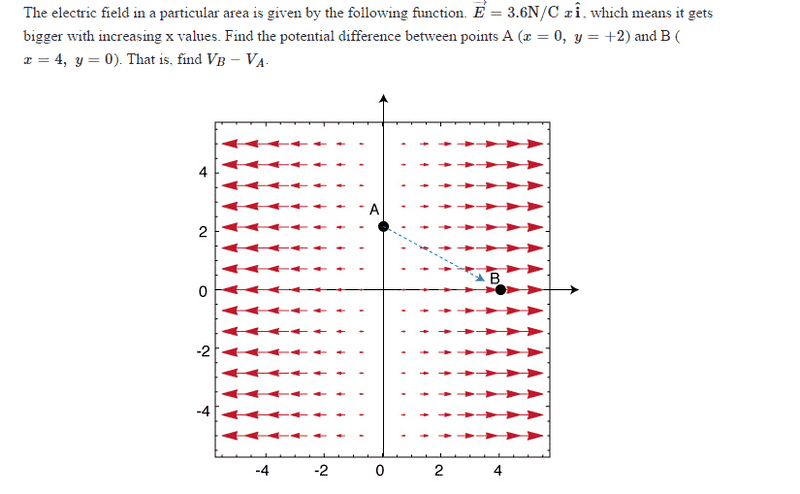# Potential difference between two points

## Homework Statement## The Attempt at a Solution

we know the electric field at A is 0, so the force is 0.

V = work / charge = U / q = F⋅D / q

the electric field at B is 4 times 3.6N/C = 14.4 N/C

since E = F/q, F = Eq

force, F = 14.4q at point b

V = 14.4q⋅d/q

the q's cancel, d is the distance from A to b which is 4.47

V = 14.4 ⋅ 4.47 = 64.39

is this correct?

Nathanael
Homework Helper
V = work / charge = U / q = F⋅D / q
"D" is the displacement, yes? This is supposed to be a vector equation, ##V=\frac{1}{q}\vec F\cdot \vec D## or just ##V=\vec E\cdot \vec D##

The other mistake is that ##V=\vec E\cdot \vec D## is not the correct equation. If the field were uniform (constant ##\vec E##), then it would be correct, but in general we must say ##V_B-V_A=\int \limits_A^B \vec E\cdot d\vec D## where the integral can be along any path from point A to point B. (##V_B-V_A## should be independent of the path from A to B, so you are free to choose whichever path seems simplest to integrate along.)

"D" is the displacement, yes? This is supposed to be a vector equation, ##V=\frac{1}{q}\vec F\cdot \vec D## or just ##V=\vec E\cdot \vec D##

The other mistake is that ##V=\vec E\cdot \vec D## is not the correct equation. If the field were uniform (constant ##\vec E##), then it would be correct, but in general we must say ##V_B-V_A=\int \limits_A^B \vec E\cdot d\vec D## where the integral can be along any path from point A to point B. (##V_B-V_A## should be independent of the path from A to B, so you are free to choose whichever path seems simplest to integrate along.)
If I take the path from A to B, should the lower and upper bounds be 0 and 4.47?

Nathanael
Homework Helper
If I take the path from A to B, should the lower and upper bounds be 0 and 4.47?
But what will you integrate with respect to? dx? dy? something else?

If you want to integrate along the straight line path, you would need to introduce a parameter. Call ##\vec A## and ##\vec B## the positions of points A and B respectively. Let's call the parameter s. Then you could parameterize the position vector of the charge like this:
##\vec R=\vec A+\frac{\vec B-\vec A}{|\vec B - \vec A|}s##.
See, ##\frac{\vec B-\vec A}{|\vec B - \vec A|}## is the unit vector from A to B. So, when you move along the parameter s, you are moving along the straight line from A to B (and the magnitude of s represents how far you've moved from A).
Then we would have
##d\vec D = \frac{\vec B-\vec A}{|\vec B - \vec A|}ds##
and then we would integrate from ##0 \text{ to }|\vec B - \vec A|## (≈4.47).

Slightly simpler would be to use the parametrization ##\vec R=\vec A+(\vec B-\vec A)s## and integrate from s=0 to s=1. (See when s=0, ##\vec R=\vec A##, and when s=1, ##\vec R= \vec B##)

You can choose any path you want though. I would suggest integrating along the path consisting of two straight lines, from (2,0) to (0,0), and then from (0,0) to (4,0). Then you can integrate with respect to dy for the first part, and w.r.t. dx for the second part.